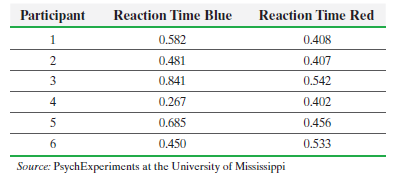×
Get Full Access to Statistics: Informed Decisions Using Data - 5 Edition - Chapter 15.4 - Problem 13
Get Full Access to Statistics: Informed Decisions Using Data - 5 Edition - Chapter 15.4 - Problem 13

×

# ?Reaction Time In an experiment conducted online at the University of Mississippi, participants are asked to react to a stimulus such as pressing a keyISBN: 9780134133539 240

## Solution for problem 13 Chapter 15.4

Statistics: Informed Decisions Using Data | 5th Edition

• Textbook Solutions
• 2901 Step-by-step solutions solved by professors and subject experts
• Get 24/7 help from StudySoup virtual teaching assistantsStatistics: Informed Decisions Using Data | 5th Edition

4 5 1 283 Reviews
19
5
Problem 13

Reaction Time In an experiment conducted online at the University of Mississippi, participants are asked to react to a stimulus such as pressing a key upon seeing a blue screen. The time to press the key (in seconds) is measured and the same person is then asked to press a key upon seeing a red screen, again with the time to react measured. The results for six study participants are shown. Does the evidence suggest that the median reaction time to the blue stimulus is different from the median reaction time to the red stimulus? Use the= 0.05 level of significance.Step-by-Step Solution:

Step 1 of 5) Reaction Time In an experiment conducted online at the University of Mississippi, participants are asked to react to a stimulus such as pressing a key upon seeing a blue screen. The time to press the key (in seconds) is measured and the same person is then asked to press a key upon seeing a red screen, again with the time to react measured. The results for six study participants are shown. Does the evidence suggest that the median reaction time to the blue stimulus is different from the median reaction time to the red stimulus Use the = 0.05 level of significance. Finding t-Values Problem Find the t-value such that the area under the t-distribution to the right of the t-value is 0.10, assuming 15 degrees of freedom (df). That is, find t0.10 with 15 degrees of freedom. Approach Step 1 Draw a t-distribution with the unknown t-value labeled. Shade the area under the curve to the right of the t-value, as in Figure 12.

Step 2 of 2

## Discover and learn what students are asking

Statistics: Informed Decisions Using Data : Inference about Two Population Standard Deviations
?In Problems 9–14, assume that the populations are normally distributed. Test the given hypothesis. 11. s1 7 s2 at the a = 0.01 level of signi

Unlock Textbook Solution